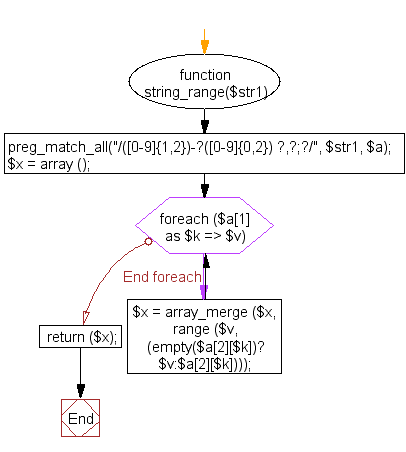﻿ PHP Array Exercise: Generate an array with a range taken from a string - w3resource# PHP Array Exercises : Generate an array with a range taken from a string

## PHP Array: Exercise-29 with Solution

Write a PHP program to generate an array with a range taken from a string.

Sample Solution:

PHP Code:

``````<?php
function string_range(\$str1)
{
preg_match_all("/([0-9]{1,2})-?([0-9]{0,2}) ?,?;?/", \$str1, \$a);
\$x = array ();
foreach (\$a as \$k => \$v)
{
\$x  = array_merge (\$x, range (\$v, (empty(\$a[\$k])?\$v:\$a[\$k])));
}
return (\$x);
}
\$test_string = '1-2 18-20 9-11';
print_r(string_range(\$test_string));
?>
```
```

Sample Output:

```Array
(
 => 1
 => 2
 => 18
 => 19
 => 20
 => 9
 => 10
 => 11
)
```

Flowchart:PHP Code Editor:

What is the difficulty level of this exercise?

﻿

## PHP: Tips of the Day

PHP: Extract numbers from a string

```\$str = 'In My Cart : 11 12 items';
preg_match_all('!\d+!', \$str, \$matches);
print_r(\$matches);
```